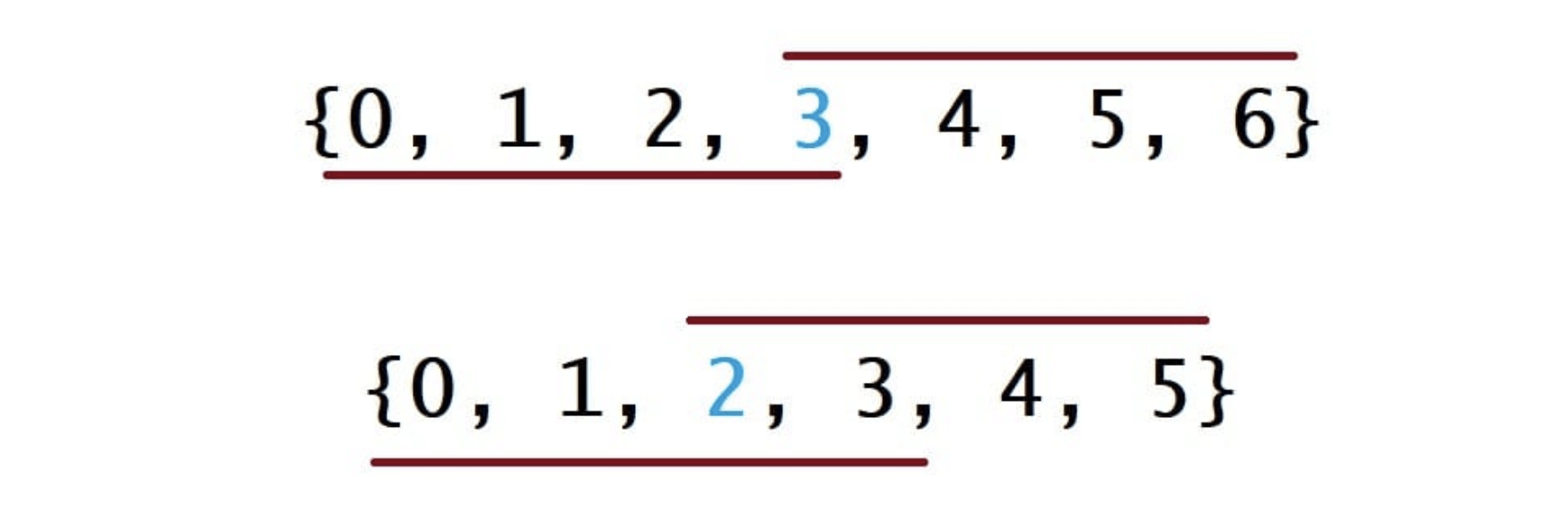# 169. Majority Element

Reference: LeetCode
Difficulty: Easy

Here is a nice article about Majority Voting Algorithm by Greg Grothaus.

## Problem

Given an array of size $n$, find the majority element. The majority element is the element that appears more than ⌊n/2⌋ times.

Note: You may assume that the array is non-empty and the majority element always exist in the array.

Example:

Follow up: Jesus. Many possible solutions.

## Analysis

### Brute-Force

Time: $O(N^2)$
Space: $O(1)$

### HashMap

It reduces the runtime to $O(N)$ with some extra space $O(N)$.

Time: $O(N)$
Space: $O(N)$

### Sorting

If the elements are sorted, the majority element is guaranteed to be at right-leaning or left-leaning mid. Think of two extreme cases when the majority element starts at the beginning or reaches the tail.Time: $O(N\log{N})$
Space: $O(1)$ (in-place) or $O(N)$ (non-destructive)

### Randomization

Because more than ⌊n/2⌋ array indices are occupied by the majority element, a random array index is likely to contain the majority element.

Note: The commented lines are not allowed (unreachable statement), since the compiler knows that the condition of the loop is true.

Time: $O(\infty)$
Space: $O(1)$

It is theoretically possible for this algorithm to r un indefinitely, so the worst possible runtime is unbounded. But in fact the expected runtime is far better which is $O(N)$.

### Divide and Conquer

Idea: If we know the majority element in the left and right halves of an array, we can determine which is the global majority element in $O(N)$.

• The base case of this problem is when the slice is length-1, and the majority element for this slice is just the only element.
• If the current slice is longer than length-1, we must combine the results from the left slice and right slice.
• If they agree on the majority element, then this element is the global majority element.
• If they disagree, we need to count the occurrences of the left and right majority elements to get the answer since we know that only one of them can be right.

Time: $T(N) = 2T(N/2) + 2N = O(N\log{N})$
Space: $O(\log{N})$

### Boyer-Moore Voting Algorithm

The idea is to count instances of the majority element as $+1$ and instances of any other element as $-1$, and then summing them would make the majority element obvious.

• We have a count variable initialized with $0$. We increment it by one whenever we see an instance of the candidate, and decrement it by one whenever we see something else.
• When count equals $0$, we effectively forget about everything before the current index. Also, we consider the element at the next index as a new candidate of the majority element.
• Eventually, a suffix will be found for which count does not hit 0.

Note: It is still possible that there is no majority element at all (e.g. 1 4 1 1 3 5 9 8 7, fake candidate is 7). So actually we need a second pass to confirm the result.

Example 1: (n = 16, #7 = 10, #5 = 5, #1 = 1)

Example 2: (n = 16, #7 = 6, #5 = 9, #1 = 1)

Note: Check if count == 0 before updating it.

Time: $O(N)$
Space: $O(1)$

CommentJunhao Wang
a software engineering cat